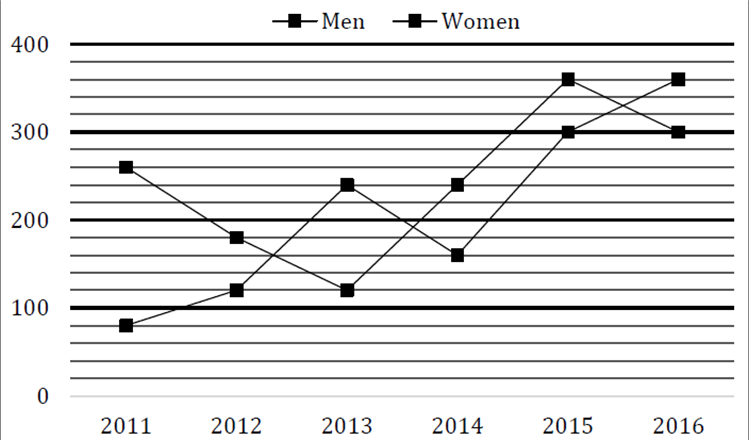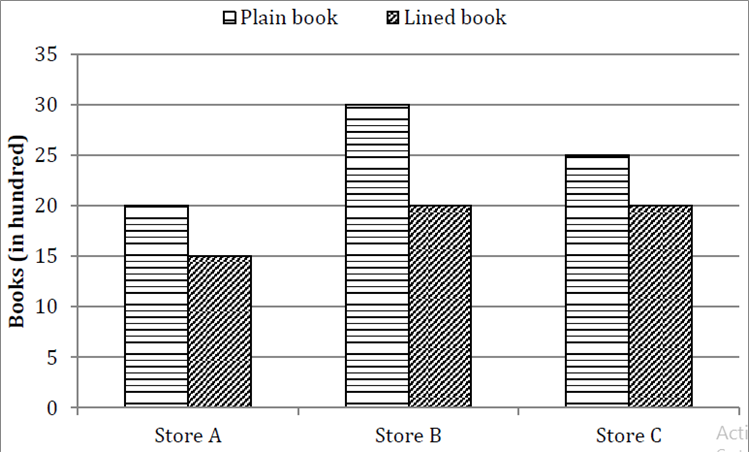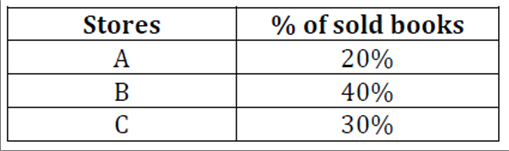# SBI Clerk Numerical Ability Online Test Questions Test 7

1.
Directions(Q.1 to Q.5 ):

Line chart given below shows number of labors (men and women) working in six different years. Study the data carefully and answer the following questions.Total number of Men working in 2012 and 2013 together is what percent of the total number of labors (Men + Women) working in 2014?

a

60%

b

70%

c

80%

d

90%

e

40%

2.

Average number of Women working in 2014, 2015 and 2016 together is how much more/less than average number of Men working in 2011, 2014 and 2016 together?

a

100

b

80

c

90

d

70

e

None of the given options

3.

Number of Men working in 2017 is 15% more than that of 2015 while number of Women working in 2017 is 40% less than that of 2014. Find total number of labors (Men + Women) working in 2017?

a

561

b

456

c

489

d

594

e

630

4.

Find the ratio between total number of Labors working in 2012 and 2013 together to total number of labors working in 2015 and 2016 together?

a

2 : 1

b

1 : 2

c

35 : 66

d

11 : 10

e

None of the given options

5.

Total number of Men working in all six years is how much more/less than total number of Women working in all six years together?

a

None of the given options

b

140

c

160

d

180

e

200

6.
Directions(Q.6 to Q.10 ):

Find the wrong number in the following number series:

4, 5.1, 7.3, 10.6, 15, 20, 27.1

a

5.1

b

4

c

7.3

d

20

e

27.1

7.

2, 3, 8, 31, 154, 924, 6460

a

924

b

6460

c

154

d

8

e

31

8.

251, 252, 254, 227, 243, 118, 154

a

251

b

252

c

227

d

243

e

154

9.

141, 156, 147, 162, 153, 165, 159

a

156

b

153

c

147

d

165

e

159

10.

2, 6, 10, 19, 36, 69, 134

a

134

b

69

c

6

d

2

e

10

11.
Directions(Q.11 to Q.21 ):

0.5, 2, 1, 4, 32, 512, 16384

a

1

b

2

c

4

d

32

e

512

12.

Ratio of present ages of A and B is 16:7. After 12 years, A’s age is twice of B’s age then find present ages of A and B?

a

64yr; 28yr

b

80yr; 35yr

c

None of these

d

96yr; 42 yr

e

102yr; 49yr

13.

A man invested a certain sum in scheme A at 15% p.a. for 2 years and earned Rs 1950 as simple interest. He increased his sum by Rs. ’x’ and invested in another scheme B at 10% p.a. C.I. for 2 years and received Rs. 1680 as compound interest. Find the value of ’x’ ?

a

Rs. 1750

b

Rs. 1500

c

Rs. 1250

d

None of these

e

Rs. 1850

14.

In a class there are 30 girls and 15 boys and total average weight of class is 47$frac{7}{{15}}$ kg. Total average weight of boys is 58 kg. Find the approximate average weight of girls ?

a

32 kg

b

42 kg

c

52 kg

d

35 kg

e

50 kg

15.

Ram bought a bike at 20% discount on MRP. After 1 year Ram sell the bike to Ramesh at 10% loss. After 1 year more Ramesh sell the bike at 20% profit to Ranjan. If Ranjan paid Rs. 1,29,600, then find the M.R.P. of the bike ?

a

1,50,000

b

2,25,000

c

1,40,000

d

2,00,000

e

1,80,000

16.
Directions(Q.16 to Q.20 ):

There are 450 coupons which can be used in Pedicure and Hair cutting. Ratio between Males to Females who use their coupons in Hair cutting is 13 : 7 Number of males who use their coupons in Pedicure is 72 more than number of females who use their coupon in Hair cutting. Total number of males who use their coupon in Pedicure and Haircutting together is 174 more than total number of females who use their coupon in Pedicure and Haircutting together.

Males who use their coupon in Pedicure is what percent of the Males who use their coupons in Haircutting?

a

200%

b

100%

c

None of the given options

d

0%

e

150%

17.

Find the ratio between Total number persons who use their coupons in Pedicure to total number of persons who use their coupons in Haircutting?

a

52 : 23

b

None of the given options

c

8 : 9

d

8 : 7

e

7 : 8

18.

Females who use their coupon in Haircutting is how much more than Females who use their coupon in Pedicure?

a

15

b

45

c

30

d

None of the given options

e

60

19.

Out of males who use their coupons in Haircutting, 25% belongs to city A, then find number of males who use their coupons in Haircutting which doesn’t belongs to city A?

a

None of the give options

b

108

c

126

d

117

e

135

20.

Ratio between Males who use their coupon in Pedicure to that of in Spa is 4 : 5, while ratio between Females who use their coupon in Haircutting to that of in Spa is 6 : 11. Find total number of people who use their coupons in Spa?

a

349

b

481

c

300

d

440

e

None of the given options

21.
Directions(Q.21 to Q.24 ):

In each of these questions, two equations (i) and (ii) are given, you have to solve both the equations and give answer accordingly.

II. $15{y^2} + 16y + 4 = 0$

a

x > y

b

x < y

c

x ≥ y

d

x ≤ y

e

x = y or no relation can be established between x & y.

22.

(i) 2x³ = √256

(ii) 2y² – 9y + 10 = 0

a

x = y or no relation can be established between x & y.

b

x < y

c

x ≤ y

d

x ≥ y

e

x > y

23.

(i) 6x² – 11x + 4 = 0

(ii) 3y² – 5y + 2 = 0

a

x ≤ y

b

x < y

c

x ≥ y

d

x > y

e

x = y or no relation can be established between x & y.

24.

(i) 3x² + 11x + 10 = 0

(ii) 2y² + 11y + 14 = 0

a

x ≥ y

b

x ≤ y

c

x > y

d

x < y

e

x = y or no relation can be established between x & y.

25.
Directions(Q.25 to Q.35 ):

(i) 12x² + 11x + 2 = 0

(ii) 12y²+ 7y + 1 = 0

a

x ≥ y

b

x = y or no relation can be established between x & y.

c

x < y

d

x ≤ y

e

x > y

26.

(i) 21x² + 10x + 1 = 0

(ii) 24y²+ 26y + 5 = 0

a

x ≤ y

b

x = y or no relation can be established between x & y.

c

x ≥ y

d

x > y

e

x < y

27.

’A’ can complete a work in 20 days while B is 25% more efficient than ’A’. B worked for 6 days and left, remaining work is completed by ’C’ in 15 days. Find in how many days ’C’ can complete the whole work alone?

a

27 days

b

21 days

c

18 days

d

24 days

e

30 days

28.

A man travels from Point P to Q with 90 km/hr and from Q to R with 60 km/hr. Total distance between P to R is 200 km. If his average speed is 75 km/hr then find the distance between P and Q?

a

80 km

b

120 km

c

100 km

d

150 km

e

None of the given options

29.

A mixture contains wine and water in the ratio 5 : 1. On adding 5 litre of water, the ratio of wine to water becomes 5 : 2. The quantity of wine in the mixture is ?

a

20 ℓ

b

22 ℓ

c

24 ℓ

d

26 ℓ

e

None of these

30.

The average salary of the entire staff in an office is Rs 3200 per month. The average salary of officers is Rs 6800 and that of non-officers is Rs 2000. If the number of officers is 5, then find the number of non-officers in the office?

a

8

b

12

c

15

d

5

e

None of these

31.
Directions(Q.31 to Q.35 ):

Given bar graph shows the number of plain books and lined books (in hundreds) available at three different stores and the table shows the percentage of total books (Plain + lined) that was sold by different stores.dataThe number of plain books sold by store A and store B was 30% and 40% respectively then find number of lined books sold by store A and store B together is what percent of total books available at store A ?

a

22 $frac{6}{7}$ %

b

23 $frac{4}{7}$%

c

25$frac{5}{7}$%

d

25%

e

None of these

32.

Average of total books sold by stores B and C together is how much more than total unsold books of store A.

a

1125

b

1075

c

1055

d

1175

e

1225

33.

Ratio of sold plain and lined books for store C is 5 : 4 and for store B is 3 : 2. Then find the total plain books sold by these two stores together?

a

1750

b

1825

c

1850

d

1950

e

1975

34.

Unsold books of store A is approximately is what percent more or less than total unsold books of store B and C together.

a

48%

b

54%

c

59%

d

52%

e

57%

35.

Selling price of each plain books and lined books sold by store B is Rs. 250 and Rs. 175 respectively. Then, find the total amount earned by store B on selling these books if 60% of lined books are sold by the store ?

a

Rs. 2.5 lac

b

Rs. 3.6 lac

c

3.5 lac

d

3.8 lac

e

4.1 lac

Rate This:
NaN / 5 - 1 votes

### SBI Clerk Numerical Ability Online Test Questions Test 7

» Your comments will be displayed only after manual approval.